$\newcommand{\NN}{\mathbb{N}} \newcommand{\CC}{\mathbb{C}} \newcommand{\GG}{\mathbb{G}} \newcommand{\LL}{\mathbb{L}} \newcommand{\PP}{\mathbb{P}} \newcommand{\QQ}{\mathbb{Q}} \newcommand{\RR}{\mathbb{R}} \newcommand{\VV}{\mathbb{V}} \newcommand{\ZZ}{\mathbb{Z}} \newcommand{\FF}{\mathbb{F}} \newcommand{\KK}{\mathbb{K}} \newcommand{\UU}{\mathbb{U}} \newcommand{\EE}{\mathbb{E}} \newcommand{\Aa}{\mathcal{A}} \newcommand{\Bb}{\mathcal{B}} \newcommand{\Cc}{\mathcal{C}} \newcommand{\Dd}{\mathcal{D}} \newcommand{\Ee}{\mathcal{E}} \newcommand{\Ff}{\mathcal{F}} \newcommand{\Gg}{\mathcal{G}} \newcommand{\Hh}{\mathcal{H}} \newcommand{\Ii}{\mathcal{I}} \newcommand{\Jj}{\mathcal{J}} \newcommand{\Kk}{\mathcal{K}} \newcommand{\Ll}{\mathcal{L}} \newcommand{\Mm}{\mathcal{M}} \newcommand{\Nn}{\mathcal{N}} \newcommand{\Oo}{\mathcal{O}} \newcommand{\Pp}{\mathcal{P}} \newcommand{\Qq}{\mathcal{Q}} \newcommand{\Rr}{\mathcal{R}} \newcommand{\Ss}{\mathcal{S}} \newcommand{\Tt}{\mathcal{T}} \newcommand{\Uu}{\mathcal{U}} \newcommand{\Vv}{\mathcal{V}} \newcommand{\Ww}{\mathcal{W}} \newcommand{\Xx}{\mathcal{X}} \newcommand{\Yy}{\mathcal{Y}} \newcommand{\Zz}{\mathcal{Z}} \newcommand{\al}{\alpha} \newcommand{\la}{\lambda} \newcommand{\ga}{\gamma} \newcommand{\Ga}{\Gamma} \newcommand{\La}{\Lambda} \newcommand{\Si}{\Sigma} \newcommand{\si}{\sigma} \newcommand{\be}{\beta} \newcommand{\de}{\delta} \newcommand{\De}{\Delta} \renewcommand{\phi}{\varphi} \renewcommand{\th}{\theta} \newcommand{\om}{\omega} \newcommand{\Om}{\Omega} \renewcommand{\epsilon}{\varepsilon} \newcommand{\Calpha}{\mathrm{C}^\al} \newcommand{\Cbeta}{\mathrm{C}^\be} \newcommand{\Cal}{\text{C}^\al} \newcommand{\Cdeux}{\text{C}^{2}} \newcommand{\Cun}{\text{C}^{1}} \newcommand{\Calt}{\text{C}^{#1}} \newcommand{\lun}{\ell^1} \newcommand{\ldeux}{\ell^2} \newcommand{\linf}{\ell^\infty} \newcommand{\ldeuxj}{{\ldeux_j}} \newcommand{\Lun}{\text{\upshape L}^1} \newcommand{\Ldeux}{\text{\upshape L}^2} \newcommand{\Lp}{\text{\upshape L}^p} \newcommand{\Lq}{\text{\upshape L}^q} \newcommand{\Linf}{\text{\upshape L}^\infty} \newcommand{\lzero}{\ell^0} \newcommand{\lp}{\ell^p} \renewcommand{\d}{\ins{d}} \newcommand{\Grad}{\text{Grad}} \newcommand{\grad}{\text{grad}} \renewcommand{\div}{\text{div}} \newcommand{\diag}{\text{diag}} \newcommand{\pd}{ \frac{ \partial #1}{\partial #2} } \newcommand{\pdd}{ \frac{ \partial^2 #1}{\partial #2^2} } \newcommand{\dotp}{\langle #1,\,#2\rangle} \newcommand{\norm}{|\!| #1 |\!|} \newcommand{\normi}{\norm{#1}_{\infty}} \newcommand{\normu}{\norm{#1}_{1}} \newcommand{\normz}{\norm{#1}_{0}} \newcommand{\abs}{\vert #1 \vert} \newcommand{\argmin}{\text{argmin}} \newcommand{\argmax}{\text{argmax}} \newcommand{\uargmin}{\underset{#1}{\argmin}\;} \newcommand{\uargmax}{\underset{#1}{\argmax}\;} \newcommand{\umin}{\underset{#1}{\min}\;} \newcommand{\umax}{\underset{#1}{\max}\;} \newcommand{\pa}{\left( #1 \right)} \newcommand{\choice}{ \left\{ \begin{array}{l} #1 \end{array} \right. } \newcommand{\enscond}{ \left\{ #1 \;:\; #2 \right\} } \newcommand{\qandq}{ \quad \text{and} \quad } \newcommand{\qqandqq}{ \qquad \text{and} \qquad } \newcommand{\qifq}{ \quad \text{if} \quad } \newcommand{\qqifqq}{ \qquad \text{if} \qquad } \newcommand{\qwhereq}{ \quad \text{where} \quad } \newcommand{\qqwhereqq}{ \qquad \text{where} \qquad } \newcommand{\qwithq}{ \quad \text{with} \quad } \newcommand{\qqwithqq}{ \qquad \text{with} \qquad } \newcommand{\qforq}{ \quad \text{for} \quad } \newcommand{\qqforqq}{ \qquad \text{for} \qquad } \newcommand{\qqsinceqq}{ \qquad \text{since} \qquad } \newcommand{\qsinceq}{ \quad \text{since} \quad } \newcommand{\qarrq}{\quad\Longrightarrow\quad} \newcommand{\qqarrqq}{\quad\Longrightarrow\quad} \newcommand{\qiffq}{\quad\Longleftrightarrow\quad} \newcommand{\qqiffqq}{\qquad\Longleftrightarrow\qquad} \newcommand{\qsubjq}{ \quad \text{subject to} \quad } \newcommand{\qqsubjqq}{ \qquad \text{subject to} \qquad }$

Image Processing with Wavelets

# Image Processing with Wavelets

This numerical tour overviews the use of wavelets for image approximation and denoising.

## Installing toolboxes and setting up the path.

You need to download the following files: signal toolbox and general toolbox.

You need to unzip these toolboxes in your working directory, so that you have toolbox_signal and toolbox_general in your directory.

For Scilab user: you must replace the Matlab comment '%' by its Scilab counterpart '//'.

Recommandation: You should create a text file named for instance numericaltour.sce (in Scilab) or numericaltour.m (in Matlab) to write all the Scilab/Matlab command you want to execute. Then, simply run exec('numericaltour.sce'); (in Scilab) or numericaltour; (in Matlab) to run the commands.

Execute this line only if you are using Matlab.

getd = @(p)path(p,path); % scilab users must *not* execute this


Then you can add the toolboxes to the path.

getd('toolbox_signal/');
getd('toolbox_general/');


## Wavelet Approximation

Image approximation is obtained by thresholding wavelets coefficients.

First we load an image $$f \in \mathbb{R}^N$$ of $$N = n_0 \times n_0$$ pixels.

name = 'cortex';
n0 = 512;
f = rescale( sum(f,3) );


Display it.

clf;
imageplot(f);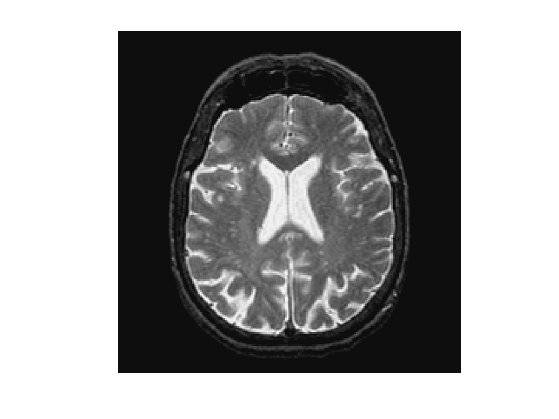An orthogonal wavelet basis $$\mathcal{B} = \{ \psi_{j,n}^k \}_{j,n}$$ of $$\mathbb{R}^N$$ is composed of multiscale atoms parameterized by their scale $$2^j$$, position $$2^j n \in [0,1]^2$$ and orentation $$k \in \{H,V,D\}$$.

A forward wavelet transform computes the set of inner products $\Psi f = \{ \langle f,\psi_{j,n}^k\rangle \} \in \mathbb{R}^N$ where the wavelet atoms are defined as $\psi_{j,n}^k(x) = \psi^k\left( \frac{x - 2^j n}{2^j} \right).$

Set the minimum scale for the transform.

Jmin = 0;


A short-cut for the wavelet transform $$\Psi$$:

Psi = @(f)perform_wavelet_transf(f,Jmin,+1);


A short-cut for the inverse wavelet transform $$\Psi^{-1} = \Psi^*$$:

PsiS = @(fw)perform_wavelet_transf(fw,Jmin,-1);


Perform the wavelet transform to compute $$\Psi f$$.

fW = Psi(f);


Display the transformed coefficients.

clf;
plot_wavelet(fW);To perform non-linear image approximation, one remove the small amplitude coefficients. This is performed using a hard thresholding $H_T(f,\mathcal{B}) = \Psi^{-1} \circ H_T \circ \Psi (f) = \sum_{|\langle f,\psi_{j,n}^k\rangle| > T} \langle f,\psi_{j,n}^k\rangle \psi_{j,n}^k.$

$$T$$ should be adapted to ensure a given number $$M$$ of non-zero coefficients, and then $$f_M = H_T(f,\mathcal{B})$$ is the best $$M$$ terms approximation of $$f$$ in $$\mathcal{B}$$.

Select a threshold.

T = .5;


Shortcut for the thresholding operator $$H_T$$.

Thresh = @(fW,T)fW .* (abs(fW)>T);


Perform hard thresholding of the coefficients.

fWT = Thresh(fW,T);


Exercice 1: (check the solution) Compute the ratio $$M/N$$ of non-zero coefficients.

exo1;

Ratio of non-zero coefficients : M/N = 0.00481.


Display the thresholded coefficients.

clf;
subplot(1,2,1);
plot_wavelet(fW);
title('Original coefficients');
subplot(1,2,2);
plot_wavelet(fWT);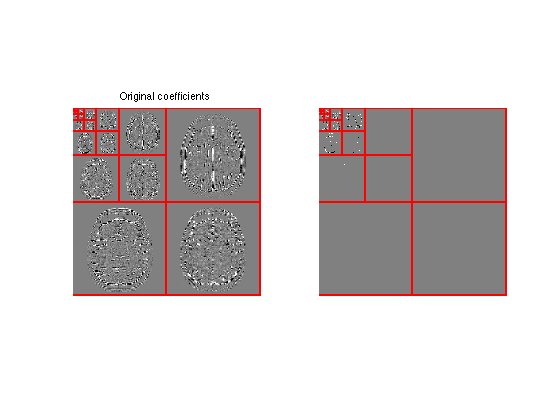Perform reconstruction using the inverse wavelet transform $$\Psi^*$$.

f1 = PsiS(fWT);


Display approximation.

clf;
imageplot(f, 'Image', 1,2,1);
imageplot(clamp(f1), strcat(['Approximation, SNR=' num2str(snr(f,f1),3) 'dB']), 1,2,2);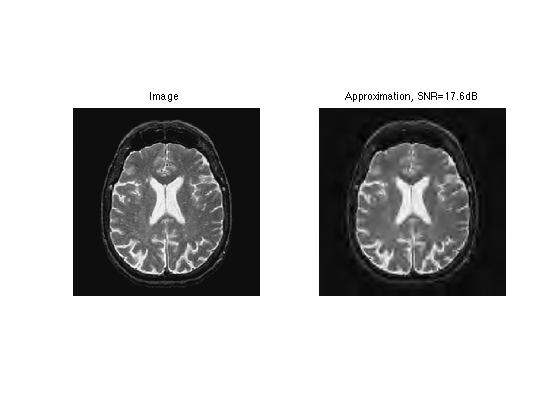Number of coefficients for the approximation.

M = n0^2/16;


Exercice 2: (check the solution) Compute a threshold $$T$$ to keep only $$M$$ coefficients.

exo2;


Perform hard thresholding.

fWT = Thresh(fW,T);


Check the number of non-zero coefficients in fWT.

disp(strcat(['      M=' num2str(M)]));
disp(strcat(['|fWT|_0=' num2str(sum(fWT(:)~=0))]));

      M=16384
|fWT|_0=16384


Exercice 3: (check the solution) Compute an approximation with an decreasing number of coefficients.

exo3;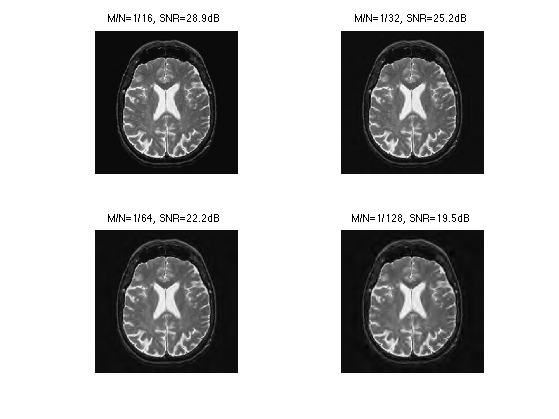## Orthognal Wavelet Denoising

Image denoising is obtained by thresholding noisy wavelets coefficielts.

Here we consider a simple setting where we intentionnaly add some noise $$w$$ to a clean image $$f$$ to obtain $$y = f + w$$.

A denoiser computes an estimate $$\tilde f$$ of $$f$$ from the observations $$y$$ alone. In the mathematical model, since $$y$$ is a random variable depending on $$w$$, so is $$\tilde f$$. A mathematical evaluation of the efficiency of the denoiser is the average risk $$E_w( \|f-\tilde f\|^2 )$$.

Here we consider a single realization of the noise, so we replace the risk by the oracle error $$\|f-\tilde f\|^2$$. This allows us to bench the efficiency of the denoising methods by comparing the result to $$f$$. But you have to keep in mind that for real application, one does not have access to $$f$$.

We consider a Gaussian white noise $$w$$ of variance $$\sigma^2$$.

sigma = .1;


Generate a noisy image.

y = f + randn(n0,n0)*sigma;


Display.

clf;
imageplot(f, 'Clean image', 1,2,1);
imageplot(clamp(y), ['Noisy image, SNR=' num2str(snr(f,y),3) 'dB'], 1,2,2);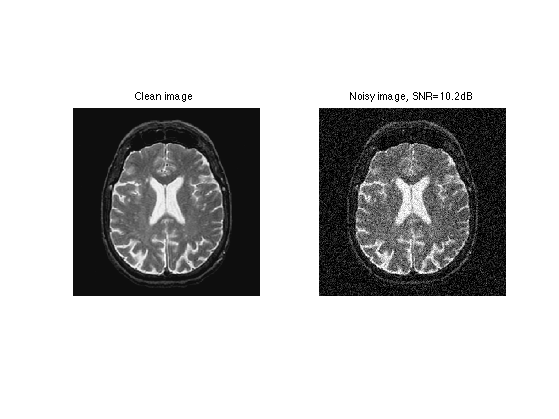A denoising is obtained by thresholding the wavelet coefficients $\tilde f = H_T(f,\mathcal{B}).$

The asymptotically optimal threshold of Donoho and Johnstone is $$T = \sqrt{2 \log(N)} \sigma$$. In practice, one observes that much better result are obtained using $$T \approx 3 \sigma$$.

Compute the noisy wavelet coefficients.

fW = Psi(y);


Compute the threshold value using the $$3\sigma$$ heuristic.

T = 3*sigma;


Perform hard thresholding.

fWT = Thresh(fW,T);


Display the thresholded coefficients.

clf;
subplot(1,2,1);
plot_wavelet(fW);
title('Original coefficients');
subplot(1,2,2);
plot_wavelet(fWT);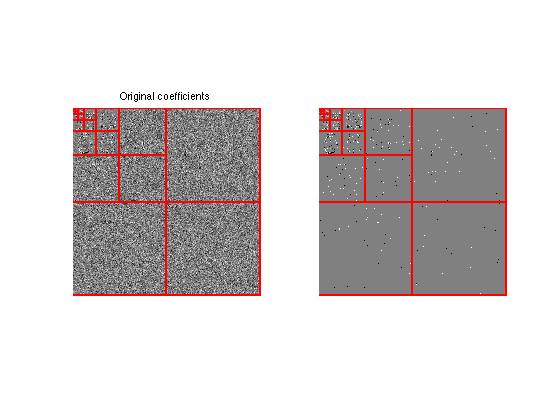Perform reconstruction.

f1 = PsiS(fWT);


Display denoising.

clf;
imageplot(clamp(y), 'Noisy image', 1,2,1);
imageplot(clamp(f1), strcat(['Denoising, SNR=' num2str(snr(f,f1),3) 'dB']), 1,2,2);Exercice 4: (check the solution) Try to optimize the value of the threshold $$T$$ to get the best possible denoising result.

exo4;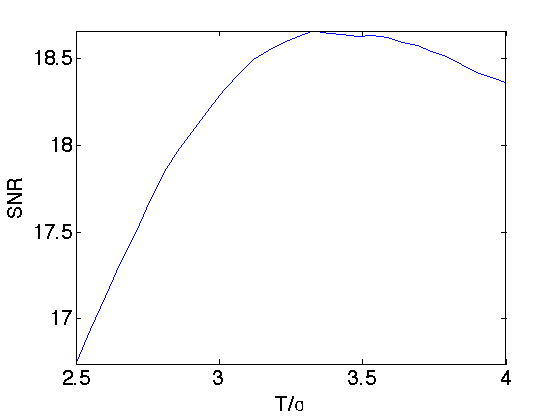## Translation Invariant Denoising

The quality of orthogonal denoising is improved by adding translation invariance. This corresponds to denoising translated copies of the image.

The translation of an image is $$(\theta_\tau f)(x) = f(x-\tau)$$, where we use periodic boundary conditions.

Given a set $$\Omega \subset \mathbb{R}^2$$, the $$\Omega$$-translation invariant denoising is defined as: $\tilde f = \frac{1}{\Omega}\sum_{\tau \in \Omega} \theta_{-\tau} \left( H_T( \theta_\tau y, \mathcal{B} ) \right).$

Here we consider translation of integer pixels in $$\{0,\ldots,\tau_{\max}-1\}$$. The number of translations is thus $$\tau_{\max}^2$$.

tau_max = 8;


Generate a set of translation vectors $$\Omega = \{ \tau_i = (X_i,Y_i) \}_i$$.

[Y,X] = meshgrid(0:tau_max-1,0:tau_max-1);


A "trick" to compute the full denoising image after all translations is to initialize $$\tilde f = 0$$, and then accumulate each denoising with translate $$\tau_i$$ in the following way: $\tilde f \longleftarrow \frac{i-1}{i} \tilde f + \frac{1}{i} \theta_{-\tau_i} \left( H_T( \theta_{\tau_i} y, \mathcal{B} ) \right)$

Initialize the denoised image $$\tilde f$$ as 0.

f1 = zeros(n0,n0);


Initialize the translation index.

i = 1;


Translate the image to obtain $$\theta_{\tau_i}(f)$$ for $$\tau_i = (X_i,Y_i)$$, with periodic boundary conditions.

fTrans = circshift(y,[X(i) Y(i)]);


Denoise this translated image, to obtain $$H_T(\theta_{\tau_i} f,\mathcal{B})$$.

fTrans = PsiS( Thresh( Psi(fTrans) ,T) );


Translate back.

fTrans = circshift(fTrans,-[X(i) Y(i)]);


Accumulate the result.

f1 = (i-1)/i*f1 + fTrans/i;


Exercice 5: (check the solution) Compute the full denoising by cycling through the $$i$$ indices.

exo5;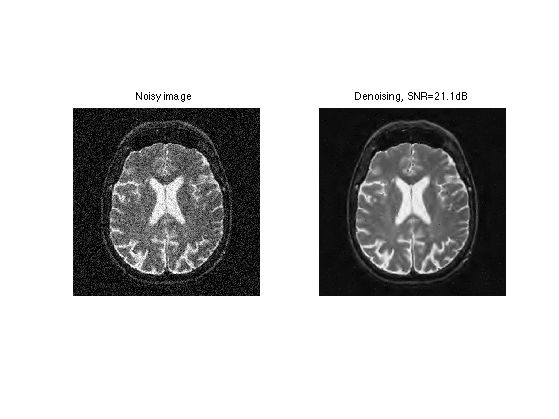Exercice 6: (check the solution) Determine the optimal threshold $$T$$ for this translation invariant denoising.

exo6;


Exercice 7: (check the solution) Test on other images.

exo7;